﻿ S-Shaped Utility Explains the Subprime Crash
Journal of Global Economics
All submissions of the EM system will be redirected to Online Manuscript Submission System. Authors are requested to submit articles directly to Online Manuscript Submission System of respective journal.
• Research Article
• J Glob Econ 2015, Vol 3(2): 146
• DOI: 10.4172/2375-4389.1000146

# S-Shaped Utility Explains the Subprime Crash

João José De Farias Neto*
Instituto de Estudos Avançados, CTA, Trevo Cel. Av. J. A. A. do Amarante, no1, Putim, São José dos Campos, SP, Brazil
*Corresponding Author: João José De Farias Neto, Instituto de Estudos Avançados, CTA, Trevo Cel. Av. J. A. A. Do Amarante, No1, Putim, São José Dos Campos, SP, Brazil, Tel: +55-(012)-3947-5315, Email: [email protected]

Received Date: Mar 09, 2015 / Accepted Date: May 08, 2015 / Published Date: May 18, 2015

### Abstract

Author propose an S-shaped utility function of consumption which, combined with a heterogeneous agents and external habit setting, it’s well the first order moments of the American financial and macroeconomic time series relevant for the equity premium puzzle in the second half of XX century. The average relative risk aversion of the agents remains in the 0-3 range. The shape of the utility and its relative risk aversion function of consumption suggest an explanation for the 2008 suprime crash.

Keywords: Financial puzzles; Subprime crash; Black swan; S-shaped utility; JEL classification: C0, C5, D1, D53, D91, E44, G12

#### Introduction

Friedman and Savage  already called attention to the puzzle that consisted on people buying lottery tickets (whose expected return is less than what is paid) and, at the same time, contracting insurance (paying more for it than the expected value of the damage); in the former case, there is a risk seeking behavior, whilst, in the later, a risk aversion; in accordance, they proposed the use of utility functions that contained local convexities. Markowitz  goes a bit further and proposes that the point that separates the convex from the concave parts of the utility be dependent upon the level of wealth. Kahneman and Tversky  proposed the so-called prospect theory. It presupposes that people make decisions based upon goals, defined by a mean and a variance of a target value; in this case, whenever the subjective probability density function of that target value is unimodal, the corresponding utility function will be S-shaped, which is explained as a predisposition of people to run risks, in order to reach a goal, and a risk aversion, when the task is to go beyond it. Another way to see it is the tendency of people to smooth their consumption time series, avoiding great oscillations, which presupposes the existence of a level with which they feel comfortable. Friedman  proposes that, due to bounded rationality, individuals don’t know their true utility function (which would be concave) and distort it, so that they end up by using an S-shaped subjective utility. The distortion would stem from the concentration of the lotteries available for him in a small range, that is, in his words, he will choose as if maximizing expected value for a value function V that in some sense is between his “true” fully considered function U and the cumulative distribution function F of prospective opportunities to increment wealth. Tummers  uses an S-shaped “welfare function of income” to analyse subjective poverty line models. The greater or smaller disposition to run risks depends upon not only the proposed lottery, but also on how many times one can play (or how many tickets you can buy); if infinite, risk neutrality is the logical consequence. That depends on the money one has: the value at risk is an important factor to be considered in investments. Worldwide, most people seem to be risk seeking, when the sum at risk is small compared to their personal wealth. Benartzi and Thaler  call attention to this kind of phenomenon, which they call myopic loss aversion and propose an S-shaped utility centered on the consumption value of the immediately preceding period (ct-1). Hamo and Heifetz  propose an explanation to the rising of spontaneous S-shaped utilities among members of a population, using evolutionary game theory. They show mathematically that society stirs its members to invest part of their resources in actuarially losing activities, because this decreases the systemic risk of the collective bet in a common direction, al-though at the expenses of the increase of the idiosyncratic risk to which the individual is exposed. The appearance of such S-shaped subjective utility functions would stem, at the individual level, from family tradition and would be collectively manifested by the dynamics of the evolutionary game between individuals with the most diverse types of utilities resulting on the gradual elimination of other kinds of utilities, so that, in the long run, 100% of the population would have that particular utility function. Ternström  posits a logistic utility of consumption, to analyze the tragedy of the commons. Neilson  says that the hypothesis that individuals base their decisions on final wealth is rejected by the data and agrees with prospect theory in that what matters are gains and losses from a reference point; he characterizes mathematically the notion of “more S-shaped than”. Levy et al.  prove that the security market line theorem of the CAPM remains valid in the context of cumulative prospect theory. Levy and Levy  show that the portfolio selected by the mean-variance approach belongs to the efficient set defined by prospect theory, whenever diversification between assets is allowed. A doubt that could arise is if this class of utilities would destroy the general equilibrium, since most of the theorems in the theory use concave utilities. Xi  proves that the operation point of each agent being beyond the point of tangency between the utility and the straight line that passes through the origin (0, 0) is a sufficient condition for the existence of the Arrow-Debreu equilibrium, when the utility is S-shaped. Hagströmer et al.  use a combination of power utilities, to build an S-shaped utility of portfolio return and show that, in this case, the full-scale optimization approach is better than the mean-variance one. Gerasymchuk  analyses cumulative normal, logistic and arctangent as possible formulas for S-shaped utilities and relates them with attitudes towards diversification of portfolios. Gerasymchuk  chooses arctangent and shows that the resulting dynamic equations for the evolution of wealth and the risky asset return exhibit chaotic regimen in a subset of its parametric space. Netzer  justifies the use of S-shaped value functions (as in prospect theory) as an evolutionary adaptation. Bostian  explores models oflearning and utility using two experimental designs and concludes that “there is some evidence of an S-shaped utility function, suggesting that risk attitudes may change for gains and losses”. Author introduce here a new utility function of consumption and argue that, as it, in a way, solves the equity premium puzzle (as is empirically shown in the paper), it can be used to explain the 2008 subprime crash. The framework of the present paper is also known as “reference-dependent expected utility”. Plus, the reference point is supposed to be the same for all agents – the per capita consumption level of the country – only changing with time. In section 2, Author introduce utility function and show some of its properties. Section 3 establishes the general framework in which the empirical work is done. Section 4 shows that the model can be narrowed. Section 5 considers the narrowed model and gets the main results. Section 6 shows results for the Brazilian market, that confirm the main conclusion obtained for the American market (namely, that my utility solves the equity premium puzzles, if, instead of considering the RRA of the average consumer, Author consider the average RRA of the consumers). Section 7 draws some conclusions and comments on the results and some of their possible implications.

#### Utility Function

Author propose that the ith household have the following utility function (cumulated modified Cauchy distribution):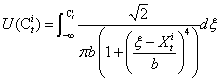(1)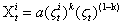(2)

a, b, κ being the same for all households, ςt being the time-smoothed mean consumption level of all the households in the country and ςt i being the time-smoothed mean consumption level of household i. Equation 2 is inspired by , who, using a different utility, gives it the flexibility of representing external and internal habit formation and combinations of both. Formula 1 has closed definite integral; using it, my utility can be expressed as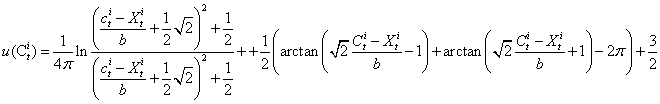(3)

In section 4, Author group sets of households by income level, using the quintiles published by the American Bureau of Labor Statistics in the period 1984-2005; thus i=1, 2, 3, 4, 5 will denote those groups of consumer units. Author use annual data. Author say that this utility generalizes and regularizes the one of Constantinides-Campbell- Cochrane for the following reasons Constantinides-Campbell- Cochrane’s utility is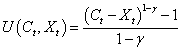(4)

If λ=2 and Xt=30, it has the graph (Figure 1). Utility 1 with Xt=30 and b=1 is plotted in (Figure 2) The difference between Figures 1 and 2 is eliminated if the right branch of the former is lifted and the vertical asymptotes are united (the resulting S-shaped graph can be shifted vertically and re-scalled to match 2). This way, (Figure 2) my utility function. The incoherence of having U(C1)>U(C2) for C1<X and C2>X, which violates one of the axioms of utility functions (the monotonicity condition), is eliminated; so is the need to use tricks to guarantee that Ct>Xt, as well as the interpretation of Xt as a subsistence level instead of habit. Abel  calls attention to this problem and proposes a utility (different from ours) that remedies it.

Worse problems occur in the left branch of utility 4 when γ is not an even integer. In the odd case, that branch is decreasing. For rational noninteger values of (for instance, 2.372-the value estimated in the 1995 version of Campbell and Cochranes paper), the left branch of U(C) is not stable under infinitesimal changes in γ, shifting from increasing to decreasing at an infinite frequency, when γ is continuously changed. For irrational values of γ, U(C)=2 R in that branch.

The functional form of the RRA, denoted here by s(C), shows the simi-larities between utilities 1 and 4 (Figure 3).

For utility 4, one has: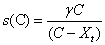(5)

For utility 1, this function is: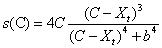(6)

So, for b=0, the RRA function of my utility is equivalent to that of my utility function of my utility is equivalent to that of utility 4 with γ=4. For b≠0, the discontinuity at C=X disappears and s(C) becomes a smooth function in R+. Now, whilst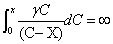and.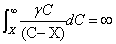my s(C) function is integrable in all of R+. Figures 3 and 4 exhibit their graphs. The absolute prudence, as defined  as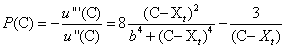(7)

It’s behavior for my utility-with a=0:92, b=0.6, κ=0 and ςt=25 is in Figure 5. It has a discontinuity on the inflexion point of the utility. From 6, it is easy to see that limC→∞ s(C)=4. The same happens in 5, for γ=4. The stochastic discount factor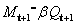(8)

where β€ (0, 1) is a subjective discount factor and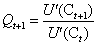(9)

is also generalized from 4 to 1, since Qt+1 corresponding to 4 is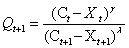(10)

while, for my utility,(11)

Equation 9 is valid here for any combination of internal and external habit, because the model is of two times (as opposed to an infinite series, which requires dynamic programming) and Author presuppose the use eqs. 16-18, that, as is mentioned there, estimates ςt as a function of {ct-2, ct-3}. In section 5, this is not needed and 9 is always valid, as  argue in their paper.

#### General Framework

Starting with a representative agent framework, Author tested my utility function in a two-times (one period) model .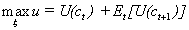(12)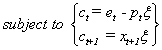(13)

where β is the subjective discount factor, et is the initial endowment that the agent has, pt is the price per share of the asset, xt+1 is the payoff per share (new price plus dividends in the period) and is the number of shares that the agent decides to buy (thus reducing his present consumption).

The well known solution, that presupposes the solution is not in a boundary, is the standard asset pricing equation: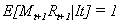(14)

where Rt+1 is the return of the investment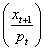, M is defined in equations 8 and 9 and It is the information set available at the moment of the decision. Subtracting equations 14 for two different assets, an equation for excess return is obtained: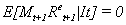(15)

where Ret+1=Rit+1 Rit+1, i and j being the assets.

Author used Fama and French’s 25 book to market portfolio and their three factors (MKT, SMB and HML) published in French’s page <http://mba.tuck.dartmouth.edu/pages/faculty/ken.french/> to estimate the parameters of my utility in equations 15. In the representative agent framework, equation 2 is reduced to t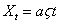. Care must be exerted, when using a habit formation utility: if the habit level çt estimation includes variables affected by the decision variable (in this case ξ), the mathematics becomes very complicated. In order to avoid this burden, Author estimate ςt using only the series {ct-2, ct-3,…}. The smoothing equations are those of the double exponential method (see the time series literature):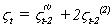(16)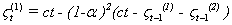(17)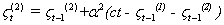(18)

Nevertheless, as the pricing equation cannot distinguish between different methods of smoothing, in many instances Author used methods that use ct; ct+1 and even c t+2;ct+3; …,like Hodrick-Prescott filter and an exponential curve adjusted to the whole consumption series (for instance: Ϛt=9e0:02(t-1950)), for reasons of convenience and/or to test the robustness of my results. As remarked in , care must be taken with respect to the time matching between returns and consumption flow. Author used NIPA 1.1.5 table (personal consumption expenditurestotal) from the US Bureau of Economic Analysis for aggregate consumption data and Frenchs site for returns; the correct matching demands a lagging in nominal time of consumption (as this is a flow, convention about when computing it-at the start or at the end of the period-varies); the CRRA utility is used to establish the correct lagging. All historical series were defeated by the CPI-U (consumer price index-urban) . Now, as conditional expectation is not available, Author use the time average of equation 15, that is, the unconditional expectation: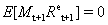(19)

The excess return of asset j is Re,j=Rj -Rf , where Rf is the return of the risk-free asset (one month t-bills). Now,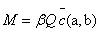where c represents the smoothing method used for the consumption series and a and b are the parameters of my utility function (see eqs 1 and 2). Instead of eq. 19, Author used eq. 20 below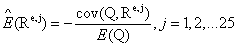(20)

to fit the model to Fama and French’s portfolio, that is, parameters (a, b) are found minimizing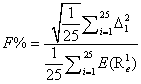(21)

where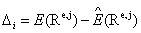Author also computed, as another fitting measure,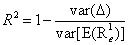(22)

Now notice that is not present in eqs. 20. It is calibrated to turn true the equation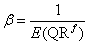(23)

In the period considered (1951-2001), the mean annual risk premium was E(Rmkt Rf )=7:87% and E(Rf )=1:22%. The mean interest rate in the period is estimated by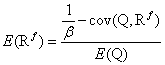(24)

using β given by equation. 23, with Q=Qc (a*, b*).

Full Model

In the full model (eqs.1 and 2), the RRA varies according to the consumption level of the agent (person or household). In order to estimate parameter, Author downloaded the quintiles of annual household expenditures series from http://www.bls.gov/ in the available period (1984-2005) and aggregated the corresponding stochastic discount factors Mi, which is allowed as a consequence of adding eqs. 19 for different consumer units: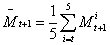(25)

To estimate (a; b; k ) , Author sought values that minimized J% as defined in 26.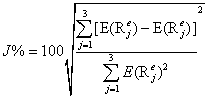(26)

In the formula above, E(Re,j) are the historical averages of the returns of Fama and French’s three factors (MKT-Rf, HML and SMB) and E (Rj) are their theoretical values using Mt+1 in the pricing equations. The results are a=0:9, b=0.2, κ=0, min J%=6.9%. Smoothed series {ζi t} were obtained by applying the Hodrick-Prescott filter with default values of the E-views software package. This establishes the model as one of pure external habit, which is also the interpretation given by Campbell and Cochrane to theirs. Figures 6-8 show the behavior of J%.

Figures 9 and 10 show the behavior of the local RRA for each quintile. In either cases, it is calculated at the average consumption level of each year of the corresponding quintile. In accordance to Figures 4 and 10 shows that the RRA at the operation point is positive for the upper two quintiles and negative for the lower two; it is also very stable, for these quintiles. The interesting discovery here is that the middle quintile appears to be psychologically bipolar, oscillating between extreme risk aversion and extreme risk seeking; this is a result of its situation making it extremely sensible to any small variation of its annual consumption.

#### External Habit

Having established, in the precedent section, that=0, Author can restrict the research to the simpler model, called in the literature external habit formation. Table of Figure 11 shows the results, using four different ways of consumption smoothing. The relatively low values of are due to the oil shocks in the period considered (1974, 1979 and 1991), which are revealed as peaks in the time series of the pricing kernel (Mt); this is how the low probability of disastrous events is internalized in my model. Estimating the parameters by applying GMM (generalized method of moments) to the Euler equations 19, with Re;j; j=MKTRF, SMB and HML (the three factors of Fama and French), Author got a=0.924 ± 0.02, b=0.59 ± 0.16 and J=0.0090. Now, recalling that the period considered was 1951-2001, we have 50J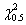, so the model passes the over identification test, that is, the three equations are not mutually incompatible. The p-values are 0.0000, for a; and 0.0005, for b. The parameters of the last line of the table in Figure 11 (which is the best fitting) were used to obtain the scatter plot of Figure 12. For comparison, points corresponding to Fama and French’s three factor models are in the graph. M FF uses the standard pricing equations E [Mt+1 Re t+1]=0, that is, with M and R contemporaneous, and pricing kernel defined by (Figure 12) Fitting to Fama and French’s portfolio.Results as good as the two models based upon the three factors of those authors.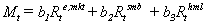(27)

Figure 12: Fitting to Fama and French’s portfolio. Results as good add the two models based upon the three factors of those authors.

where Rte;mkt=Rt mkt Rtf (all R’s taken from French’s page). FF regres uses the arbitrage regression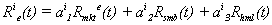(28)

i=1,2,...,25, which has 75 free parameters estimated by ordinary least squares. Figures 13 and 14 show the best fitting of power and recursive utility to Fama and French’s portfolio. Their pricing capabilities are visibly poorer than the one of my utility function. The RRA calculated at the per capita consumption level (that is, at the representative agent’s level) is exhibited. Notice how its mean level is about the same as the one of Campbell and Cochrane. Thus, in the representative agent framework, the equity premium puzzle persists; the risk free puzzle disappeared, since both those authors and us succeeded in explaining the historical mean interest rate with β ϵ (0, 1) (in my case, β ≈ 0.5 , as shown in the table Figure 11). Figures 15 and 16 show the situation for 2004: the representative agent operates at the edge.

Figure 14: The best fitting of Epstein-Zin-Weil utility that allowsß<1: RRA=87. b=0.84 = E (Rf)=1.22%. F%=31%. R2=0.35.

Figure 15: The operation point is near the edge of the cliff. The Graph shows that,in 2004, Xi’s condition is satisfied. Since the operation point is beyond the tangency point.

Main result

Dragulesco and Yakovenko  show that the cross-sectional distribution of income in US can be well modelled by the usual exponential; Husby  exhibits a linear equation relating consumption with income:

C = 3.64 + 0:9016Y    (29)

Equation. 29 is expressed in aggregate values of 1961; in per capita values, using dollars of the year 2000, the intercept 3.64 represents about US\$400 per year, against a per capita consumption of about US\$10,000 in 1961. Thus, the intercept can be despised and Author can adopt the exponential distribution for consumption too, that is: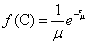(30)

Where μ=c=per capita annual consumption.

Average RRA: Proposition: Suppose, in the heterogeneous case, that each consumer i have a power utility of consumption, that is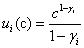(31)

and that the resulting collective utility be the geometric average of the individual utilities, that is: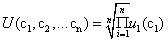(32)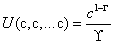(33)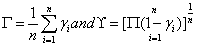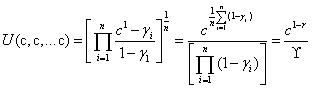Where (Figure 17) Q.E. D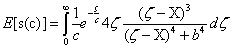(34)

This proposition shows that the average of the RRAs has some theoretical significance.

Averaging the RRA function s(C) over the consumption distribution, that is, taking leads to the graphs o (Figure 17). The average RRA remains in the (0,3) interval! Considering the intercept, the consumption distribution becomes a shifted exponential and, according to my simulations, the average RRA drops a little. Notice the robustness of (Figure 18) RRA of the representative agent. The main result with respect to the smoothing method used to estimate the habit level, thus showing that the exact form of equations 16-18 is not very important; the same can be said about the general level of risk aversion at the consumption level of the representative agent: Figure 18 shows its robustness relative to the smoothing equations. Figure 19 shows that the operating point of the representative agent oscillates around Xi’s threshold.

Figure 19: Consumption of the representative agent over Xi’s threshold Ctg when C.Ctg, the general equilibrium is guaranteed to exist.

#### Comparison with other Utility Functions

Author compared this utility with the most commonly used in the literature and some other S-shaped ones. None has played so well. The table in Figure 20 shows it. M FF and FF regres are the methods used by Fama and French; Gamma is the cumulative distribution of the gamma random variable (it fits well the portfolio, but its average RRA has an upward trend). Logistic and the original Cauchy cumulative distribution were tested too; the former has a linear trend in its average RRA; the latter is not so good as Cauchy4 and one of its parameters isn’t not statistically significant.of course, an exaustive search for alternative utilities is impossible, but the sample analised singularizes the Cauchy4 as very special in many respects.

If there is a theoretical reason for that is an open matter for future research. The fact that  has chosen arctangent after examining several other candidates is more evidence in favor of the Cauchy 4, since its kernel is the arctangent function (see eq. 3).

#### Conclusion

Although a large proportion of the households (~60%)3 operates in the risk-seeking region (below the external habit level, trying to “catch up with the joneses”), the representative agent is risk-averse and operates above the habit level X=aζ most of the time, thus guaranteeing the general equilibrium [26-29]. The mix between risk-seeking and risk-averse agents is such that, as a whole, the economy can still find its equilibrium. As U(C) is the utility of annual consumption, its convexity below the habit level only means that agents operating in that region prefer to throw a coin and, next year, consume c+ε, if it turns out head, or c-ε”, if it turns out tail (ε” small compared to c), instead of a guaranteed consumption of c. It doesn’t mean that they would behave like this when confronted with any lottery offering immediate payoff of money or other benefit. They are trying to catch up with the long run standard of living of the representative agent, not necessarily seeking immediate rewards, although it is a known fact that the lower classes tend to buy (actuarially disadvantageous) lottery tickets more frequently than the upper classes. Due to the convexity of the lower part of the utility function, consumers who operate in that part and are not close enough to the per capita consumption level maximize their utility not by satisfying the first order condition (eq. 14), but by restricting their consumption at t=0 to the subsistence minimum and investing all the rest, that is, for them the subsistence level is binding. They are willing to accept any amount of credit they are offered, in order to rise from the convex to the concave part of the utility, thus attaining the average consumer level (“catching up with the joneses”). Figures 4 and 5 suggest that, as people are born and progressively have their income raised, they behave like waves that hit a beach (the central bump), thus prone to breaking and generating turbulence and, as a consequence, financial bubbles; notice that this wouldn’t happen with the usual power utility (CRRA), whose RRA function is a constant horizontal line. Thus, pressure from the risk-seeking new generation driving the government to support bad credit loans via Fannie Mae and Freddy Mac may be the ultimate responsible for the crash. The RRA function of my utility corresponds to what is observed in animal psychology: coming from higher to lower consumption values, the representative agent is first taken by panic (increased RRA), then despair (negative RRA) and, thus, disposition to risk everything, and finally desolation (negative RRA, but small in module) [26-29]. The implied fact, by the final model (external habit), that 60% of the population has negative RRA, may be an explanation for the high debt levels that this extract is willing to take, which, by its turn, could explain phenomena like the so-called “credit feast” in Brazil and the “real state credit crunch” in US. The model economy of this paper works at the edge of chaos (in a loose sense), since the operating point of the representative agent oscillates around Xi’s threshold for the existence of equilibrium, with the two points being statistically indistinguishable (the sample average of their difference is within one standard deviation from zero). The shape of the RRA function (Figure 16) hints at an explanation for conflicts in general. The parcel of the population immediately below the habit level is extremely risk-seeking, while the parcel immediately above it is extremely risk-averse; as a consequence, the former is willing to confront the latter, in order to climb the clif (Figure 15) and get to the plateau.

#### References

Citation: Farias Neto JJ (2015) S-Shaped Utility Explains the Subprime Crash. J Glob Econ 3:146. Doi: 10.4172/2375-4389.1000146

Copyright: © 2015 Farias Neto JJ. This is an open-access article distributed under the terms of the Creative Commons Attribution License, which permits unrestricted use, distribution, and reproduction in any medium, provided the original author and source are credited.

Select your language of interest to view the total content in your interested language

##### Recommended Journals
Viewmore
###### Article Usage
• Total views: 12274
• [From(publication date): 7-2015 - Aug 25, 2019]
• Breakdown by view type
• HTML page views: 8422
• PDF downloads: 3852

## Post your commentCan't read the image? click here to refresh
###### Peer Reviewed Journals

Make the best use of Scientific Research and information from our 700 + peer reviewed, Open Access Journals

International Conferences 2019-20

Meet Inspiring Speakers and Experts at our 3000+ Global Annual Meetings

Top